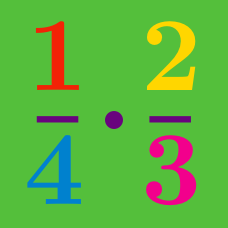Basic Mathematics

# Complex Fractions

Which of the following is equal to

$\frac { 4 + \frac{ 1 } { 4 } } {4 }?$

Which of the following is equal to

$\frac {1 }{ \left( \frac{1}{7}\right)}?$

Which of the following is equal to

$\frac { 2 + \frac{ 1 } { 8 } } {3 + \frac{ 2 } { 8 }}?$

$\frac{83}{13} = a + \frac{1}{ b + \dfrac{1}{ c + \dfrac{1}{ d + \dfrac{1}{2} } } }$

The above equation holds for positive integers $$a, \: b, \: c, \: \text{and} \: d$$.

Find $$a+b+c-d$$.

Which of the following is equal to

$\frac{3}{\dfrac{1}{2} + \dfrac{2}{3} - \dfrac{1}{4}}?$

×